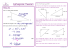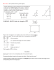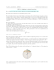# HW 1 - Solutions to selected exercises

## Transcription

HW 1 - Solutions to selected exercises
```Classical geometries - MAT402
Instructor: Ester Dalvit
HW 1 - Solutions to selected exercises
Ex 2. Given a segment of length l, explain how to construct using straight edge and compass:
√
• a rectangle with sides of length l and 2l;
• a rectangle with sides of length l and l/3.
You may use constructions of Exercise 1.
Let AB be a segment of length l. Construct a square on AB (as you should have done in exercise 1: draw
perpendiculars to AB through A and through B, draw circles of radius l and centers A and B, intersect the
circles with the perpendiculars and choose two points C, D on the same side of the line through A and B.
These are the √
other vertices of a square of side AB).
Now |AC| = l 2 by Pythagora’s theorem on triangle ABC.
Draw a circle with center A and radius AC and call E its intersection with the line AD.
Draw the perpendicular to AD through E. Let F be its intersection with line BC.
ABF E is a rectangle as requested.
Sketch of part 2: use the division of a segment into n equal parts as shown in the textbook (in our case you
may divide AD into 3 parts).
√
Remark:
|AF | = l 3 by Pythagora’s theorem.√So we can construct a right triangle with legs of length l
√
and l 3.
√ The hypothenuse will then measure l 4. And so on, inductively, we can construct segments of
length l n for any n ∈ N.
Ex 4. Check that the bisection of a line segment and construction of perpendiculars using a straightedge
and compass which were described in class actually produce the desired result.
The construction we saw is the following: given segment AB, we draw the circles with radius |AB| and
centers A and B respectively. The circles intersect in points C and D. Draw the line through C and D. It
intersects AB in point M .
Claim 1: |AM | = |BM |.
2
Claim 2: CD is perpendicular to AB.
The segments AC, AD, and AB are congruent because radii of same circles. Same for BC, BD and AB.
4BCD is isosceles (|BC| = |BD|) so ∠BCD = ∠BDC.
4ACD is congruent to 4BCD by SSS (CD is common side, all other sides are congruent).
Thus |CM | = |DM | and ∠ACD = ∠BCD = ∠ADC = ∠BDC.
4ACM in congruent to 4BCM by SAS (CM in common, |AB| = |AC|, ∠ACD = ∠BCD).
Hence |AM | = |BM | and claim 1 is proved.
Also from the congruence of the two triangle it follows that ∠AM C = ∠BM C, and since ∠AM C = ∠BM D
and ∠BM C = ∠AM D (opposite angles) then all four angles are equal. Since they sum up to 2π, any of
them is π/2. This proves claim 2.
Ex 7. Prove the converse of Pythagora’s theorem.
Let a = |BC|, b = |AC| and c = |AB| as in the picture. We know that b2 + c2 = a2 , we have to prove that
the angle in A is right.
Construct a triangle with two sides of length b and c respectively and the angle between them of π/2. Let d
be the length of the third side. Then by Pythagora’s theorem d2 = b2 + c2 .
Thus a2 = d2 and thus a = d (since both numbers are positive since they are lengths).
Then the two triangles are congruent by SSS. Hence the angle in A is right.
```

### pg. 485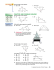### Practice Worksheet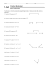### Reteach Sec 1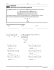### class vii holidays homework - Raja Ram Mohan Roy Public School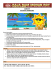### Some Ways to Prove Triangles Congruent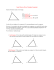### 12-2 Practice Chords and Arcs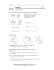### Notes 19 - Proving Triangles Congruent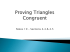### Extra-Curricular Activities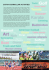### LitCircles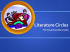### Litchfield Technology Symposium June 10 and 11 Allison Arndt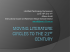### Geometry Practice Quiz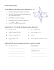### Literacy circles: Engaging reluctant learners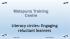### Document 6564469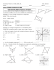### G.C.2 Wkst 5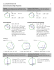### END OF COURSE GEOMETRY CORE 1 VIRGINIA STANDARDS OF LEARNING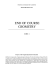### See details. - Math Awareness Month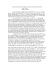### Theorem Toolkit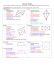### 4-3 Triangle Congruence by ASA and AAS Vocabulary### HSM12CC_GM_06_02_CM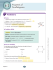### Pythagoras’ Theorem Now have a go yourself .. . .